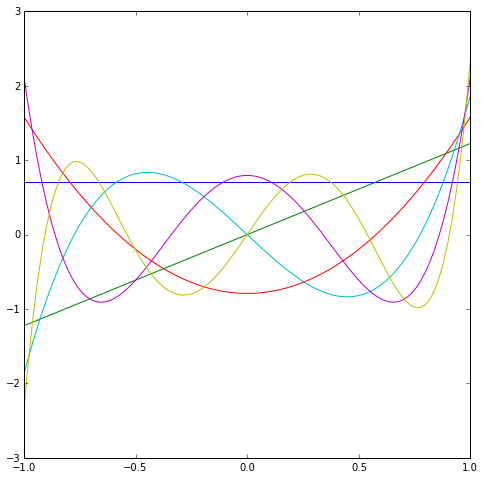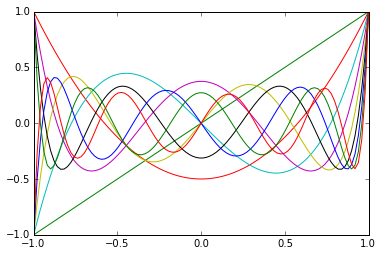# Orthogonal polynomials

In :
import numpy as np
import numpy.linalg as la
import matplotlib.pyplot as pt


## Mini-Introduction to sympy

In :
import sympy as sym

# Enable "pretty-printing" in IPython
sym.init_printing()


Make a new Symbol and work with it:

In :
x = sym.Symbol("x")

myexpr = (x**2-3)**2
myexpr

Out:
$$\left(x^{2} - 3\right)^{2}$$
In :
myexpr = (x**2-3)**2
myexpr
myexpr.expand()

Out:
$$x^{4} - 6 x^{2} + 9$$
In :
sym.integrate(myexpr, x)

Out:
$$\frac{x^{5}}{5} - 2 x^{3} + 9 x$$
In :
sym.integrate(myexpr, (x, -1, 1))

Out:
$$\frac{72}{5}$$

## Orthogonal polynomials

Now write a function inner_product(f, g):

In :
def inner_product(f, g):
return sym.integrate(f*g, (x, -1, 1))


Show that it works:

In :
inner_product(1, 1)

Out:
$$2$$
In :
inner_product(1, x)

Out:
$$0$$

Next, define a basis consisting of a few monomials:

In :
#basis = [1, x, x**2, x**3]
basis = [1, x, x**2, x**3, x**4, x**5]


And run Gram-Schmidt on it:

In :
orth_basis = []

for q in basis:
for prev_q in orth_basis:
q = q - inner_product(prev_q, q)*prev_q
q = q / sym.sqrt(inner_product(q, q))

orth_basis.append(q)

In :
orth_basis

Out:
$$\begin{bmatrix}\frac{\sqrt{2}}{2}, & \frac{\sqrt{6} x}{2}, & \frac{3 \sqrt{10}}{4} \left(x^{2} - \frac{1}{3}\right), & \frac{5 \sqrt{14}}{4} \left(x^{3} - \frac{3 x}{5}\right), & \frac{105 \sqrt{2}}{16} \left(x^{4} - \frac{6 x^{2}}{7} + \frac{3}{35}\right), & \frac{63 \sqrt{22}}{16} \left(x^{5} - \frac{10 x^{3}}{9} + \frac{5 x}{21}\right)\end{bmatrix}$$

These are called the Legendre polynomials.

What do they look like?

In :
mesh = np.linspace(-1, 1, 100)

pt.figure(figsize=(8,8))
for f in orth_basis:
f = sym.lambdify(x, f)
pt.plot(mesh, [f(xi) for xi in mesh])These functions are important enough to be included in scipy.special as eval_legendre:

!! Careful: The Scipy versions do not have norm 1.

In :
import scipy.special as sps

for i in range(10):
pt.plot(mesh, sps.eval_legendre(i, mesh))What can we find out about the conditioning of the generalized Vandermonde matrix for Legendre polynomials?

In :
n = 20
xs = np.linspace(-1, 1, n)
V = np.array([
sps.eval_legendre(i, xs)
for i in range(n)
]).T

la.cond(V)

Out:
$$7274.59818549$$